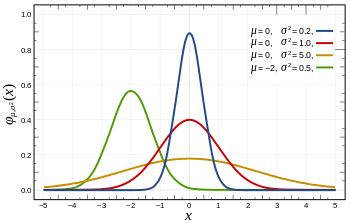# Uncertainty Wednesday: Continuous Random Variables

So far in Uncertainty Wednesdays we have only dealt with models and random variables that had a discrete probability distribution. Often in fact we had only two possible states or signal values. There are lots of real world problems though in which the variable of interest can take on a great many values. For example the time between two events taking place. We could try to break this down into discrete small intervals (say seconds) and have a probability per second. Or we could define a continuous random variable where the wait time can be any real number from some continuous range.

Now if you have been following along with this series you will have one immediate objection: how can we assign a probability to our random variable taking on a specific real number from a range? A range of reals contains uncountably infinitely many real numbers and hence thefor any single real value must be, well, infinitely small? So how do we define a Prob(X = x)?

Before I get to the answer let me interject a bit of philosophy. There is a fundamental question about the meaning of real numbers: are they actually real, as in, do they exist? OK, so this is a flippant way of asking the question. Here is a more precise way. Is physical reality continuous or quantized? If it is quantized, then using a model with real numbers is always an approximation of reality. My reading of physics is that we don’t really know the answer. A lot of phenomena are quantized but then there is something like time, which we understand extremely poorly (which is why I chose time as opposed to say distance as my example above). Personally, while not, ahem certain, I am more inclined to see real numbers as a mathematical ideal, which approximates a quantized reality.

Does this matter? Well, it does because too often continuous random variables are treated as some kind of ground truth, instead of an approximation to a physical process. And as we will see in some future Uncertainty Wednesday, often this is a rather restrictive approximation.

Now back to the question at hand. How do we define a probability for a continuous random variable? The answer is through a so-called probability density function (PDF). I find it easiest to think of the PDF as specifying the probability “mass” for an infinitesimal interval around a specific value. Let’s call our density function f(x), then the value of f(x) at x is not the probability of X = x but rather the probability of x – ε ≤ X ≤ x + ε for an infinitesimal ε (I will surely get grief from someone for this abuse of notation).

But by thinking about it this way it then follows quite readily that we can find the Probability of X being in a range by forming the integral of the probability density function for that range

Probably the single best known probability density function is the one that gives us a random variable with a Normal Distribution. The shape of the PDF is why the Normal Distribution is also often referred to as the “Bell Curve”

Next Uncertainty Wednesday we will dig a bit deeper into continuous random variables by comparing them to what we have learned about discrete ones.